# Index of /ag/schulze/Hermitian-lattices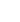NameLast modifiedSizeDescription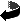Parent Directory  -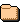D-3/12-Mar-2003 15:54 -D-4/12-Mar-2003 15:54 -D-7/12-Mar-2003 15:54 -D-8/12-Mar-2003 15:54 -D-11/12-Mar-2003 15:53 -D-15/12-Mar-2003 15:53 -D-19/12-Mar-2003 15:53 -D-20/12-Mar-2003 15:53 -D-23/12-Mar-2003 15:53 -D-24/12-Mar-2003 15:54 -D-31/12-Mar-2003 15:54 -D-35/12-Mar-2003 15:54 -D-39/12-Mar-2003 15:54 -D-40/12-Mar-2003 15:54 -D-43/12-Mar-2003 15:54 -D-56/12-Mar-2003 15:54 -D-67/12-Mar-2003 15:54 -D-163/12-Mar-2003 15:53 -D-455/12-Mar-2003 15:54 -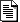README.html09-Sep-2019 19:01 5.9K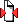hermitianlattices.tgz16-Apr-2018 09:58 15Mnoindex.html11-Mar-2004 10:33 5.5Koldindex.html16-Apr-2018 10:02 5.7K

explanation of the hermitian lists These tables have been computed by Alexander Schiemann when he was a member of my group. He also wrote the explaining text below.
If you use the results available here please quote him (not me) as the author, along with the article mentioned at the bottom of this page that explains the algorithm used.
This Webpage is currently maintained by me.

Rainer Schulze-Pillot (for email adress go up one level to the homepage of my group).

The subdirectories contain complete classifications of some genera of unimodular hermitian lattices in a hermitian space (V,h) over a complex quadratic field K=Q(sqrt(d)) .
Each subdirectory D-x contains genera in spaces over one field with discriminant -x. In these directories each file holds one genus of unimodular lattices. All classes (with respect to the unitary group U(V,h) ) are listed. Files >50K are zipped with gzip. Unimodular genera of the same dimension in different hermitian spaces are distinguished by extensions .1, .2,... Even and odd genera in the same space have an additional extension .even/.odd (only spaces of even dimension over a field with even discriminant may contain even unimodular lattices).

Notation:
In each subdirectory w denotes a member of the "standard" Z-base of the ring of integers of K=Q(sqrt(d))
where w=sqrt(d)  if d=2,3 (mod 4) or w=(1+sqrt(d)) /2  if d=1 (mod 4).
The lattices are not necessarily free modules over the ring of integers. Nevertheless, a lattice L in an n-dimensional hermitian space admits a representation
L= a_1*x_1+...+a_n*x_n
where a_i are ideals in the ring of integers and x_i vectors of a K-base of V. The lattice L is given as a list of the ideals a_1... a_n and the lower triangle of the Gram matrix with respect to x_1... x_n . The list of ideals is omitted if all ideals are the whole ring of integers. The K-base x_1... x_n is called a pseudo-base of L and the ideal a_i the coefficient ideal of L at x_i.

example:
&Hlattice (#1 <-- #8)       the 1st lattice of a list
13                       lower triangle of its Gram matrix
3-6w    16                   with respect to a base x_1,..,x_n
|Aut| = 2^2*3              order of the group of automorphisms
#short vectors:
0  0  12  12              numbers of integral vectors representing 1,2,3...

&Hlattice (#7 <-- #9)       the 7-th lattice of a list
<1> <2,-1+w>              list of ideals a_1...a_n
ideals are given by their Z-generators or <1>
for the whole ring of integers
2                         lower triangle of Gram matrix
1/2w    1                 note: 1/2w = (1/2)w
|Aut| = 2^2*3
#short vectors:
0  6  0  6

note: lattices that are free modules over the ring of integers of K may also be given in a "non-free representation" if such a pseudo-base leads to a Gram matrix with smaller coefficients. For more information see

A. Schiemann: Classification of hermitian forms with the neighbour method, J. Symbolic Comput. 26 (1998), no. 4, 487--508.

email: aschiem@math.***.de   (with ***=uni-sb)

Verantwortlich: Rainer Schulze-Pillot-Ziemen, Universität des Saarlandes, Saarbrücken
Impressum
Datenschutzhinweis